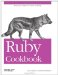# Comparing Floating-Point Numbers

Problem

Floating-point numbers are not suitable for exact comparison. Often, two numbers that should be equal are actually slightly different. The Ruby interpreter can make seemingly nonsensical assertions when floating-point numbers are involved:

```	1.8 + 0.1 # => 1.9
1.8 + 0.1 == 1.9 # => false
1.8 + 0.1 > 1.9 # => true
```

You want to do comparison operations approximately, so that floating-point numbers infintesimally close together can be treated equally.

Solution

You can avoid this problem altogether by using BigDecimal numbers instead of floats (see Recipe 2.3). BigDecimal numbers are completely precise, and work as well as as floats for representing numbers that are relatively small and have few decimal places: everyday numbers like the prices of fruits. But math on BigDecimal numbers is much slower than math on floats. Databases have native support for floating-point numbers, but not for BigDecimals. And floating-point numbers are simpler to create (simply type 10.2 in an interactive Ruby shell to get a Float object). BigDecimals can't totally replace floats, and when you use floats it would be nice not to have to worry about tiny differences between numbers when doing comparisons.

But how tiny is "tiny"? How large can the difference be between two numbers before they should stop being considered equal? As numbers get larger, so does the range of floating-point values that can reasonably be expected to model that number. 1.1 is probably not "approximately equal" to 1.2, but 1020 + 0.1 is probably "approximately equal" to 1020 + 0.2.

The best solution is probably to compare the relative magnitudes of large numbers, and the absolute magnitudes of small numbers. The following code accepts both two thresholds: a relative threshold and an absolute threshold. Both default to Float::EPSILON, the smallest possible difference between two Float objects. Two floats are considered approximately equal if they are within absolute_epsilon of each other, or if the difference between them is relative_epsilon times the magnitude of the larger one.

```	class Float
def approx(other, relative_epsilon=Float::EPSILON, epsilon=Float::EPSILON)
difference = other - self
return true if difference.abs <= epsilon
relative_error = (difference / (self > other ? self : other)).abs
return relative_error <= relative_epsilon
end
end

100.2.approx(100.1 + 0.1) # => true
10e10.approx(10e10+1e-5) # => true
100.0.approx(100+1e-5) # => false
```

Discussion

Floating-point math is very precise but, due to the underlying storage mechanism for Float objects, not very accurate. Many real numbers (such as 1.9) can't be represented by the floating-point standard. Any attempt to represent such a number will end up using one of the nearby numbers that does have a floating-point representation.

You don't normally see the difference between 1.9 and 1.8 + 0.1, because Float#to_s rounds them both off to "1.9". You can see the difference by using Kernel#printf to display the two expressions to many decimal places:

```	printf("%.55f", 1.9)
# 1.8999999999999999111821580299874767661094665527343750000
printf("%.55f", 1.8 + 0.1)
# 1.9000000000000001332267629550187848508358001708984375000
```

Both numbers straddle 1.9 from opposite ends, unable to accurately represent the number they should both equal. Note that the difference between the two numbers is precisely Float::EPSILON:

```	Float::EPSILON # => 2.22044604925031e-16
(1.8 + 0.1) - 1.9 # => 2.22044604925031e-16
```

This EPSILON's worth of inaccuracy is often too small to matter, but it does when you're doing comparisons. 1.9+Float::EPSILON is not equal to 1.9-Float::EPSILON, even if (in this case) both are attempts to represent the same number. This is why most floating-point numbers are compared in relative terms.

The most efficient way to do a relative comparison is to see whether the two numbers differ by more than an specified error range, using code like this:

```	class Float
def absolute_approx(other, epsilon=Float::EPSILON)
return (other-self).abs <= epsilon
end
end

(1.8 + 0.1).absolute_approx(1.9) # => true
10e10.absolute_approx(10e10+1e-5) # => false
```

The default value of epsilon works well for numbers close to 0, but for larger numbers the default value of epsilon will be too small. Any other value of epsilon you might specify will only work well within a certain range.

Thus, Float#approx, the recommended solution, compares both absolute and relative magnitude. As numbers get bigger, so does the allowable margin of error for two numbers to be considered "equal." Its default relative_epsilon allows numbers between 2 and 3 to differ by twice the value of Float::EPSILON. Numbers between 3 and 4 can differ by three times the value of Float::EPSILON, and so on.

A very small value of relative_epsilon is good for mathematical operations, but if your data comes from a real-world source like a scientific instrument, you can increase it. For instance, a Ruby script may track changes in temperature read from a thermometer that's only 99.9% accurate. In this case, relative_epsilon can be set to 0.001, and everything beyond that point discarded as noise.

```	98.6.approx(98.66) # => false
98.6.approx(98.66, 0.001) # => true
```

• Recipe 2.3, "Representing Numbers to Arbitrary Precision," has more information on BigDecimal numbers
• If you need to represent a fraction with an infinite decimal expansion, use a Rational number (see Recipe 2.4, "Representing Rational Numbers")
• "Comparing floating-point numbers" by Bruce Dawson has an excellent (albeit C-centric) overview of the tradeoffs involved in different ways of doing floating-point comparisons (http://www.cygnus-software.com/papers/comparingfloats/comparingfloats.htm)Ruby Cookbook (Cookbooks (OReilly))
ISBN: 0596523696
EAN: 2147483647
Year: N/A
Pages: 399